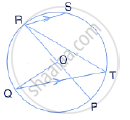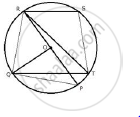Share

The Given Figure Shows a Circle with Centre O Such that Chord Rs is Parallel to Chord Qt, Angle Prt = 20° and Angle Poq = 100°. Calculate: (Iv) Angle Str - ICSE Class 10 - Mathematics

ConceptArc and Chord Properties - If Two Arcs Subtend Equal Angles at the Center, They Are Equal, and Its Converse

Question

The given figure shows a circle with centre O such that chord RS is parallel to chord QT, angle PRT = 20° and angle POQ = 100°. Calculate:

(iv) angle STRSolutionJoin PQ, RQ and ST.

Since RSTQ is a cyclic quadrilateral
∴ ∠QRS + ∠QTS = 180° (sum of opposite angles)
⇒  ∠QRS + ∠QTS + ∠STR = 180°
⇒ 110 + 40  + ∠STR =  180°
⇒ ∠STR =  30°

Is there an error in this question or solution?

APPEARS IN

Solution The Given Figure Shows a Circle with Centre O Such that Chord Rs is Parallel to Chord Qt, Angle Prt = 20° and Angle Poq = 100°. Calculate: (Iv) Angle Str Concept: Arc and Chord Properties - If Two Arcs Subtend Equal Angles at the Center, They Are Equal, and Its Converse.
S